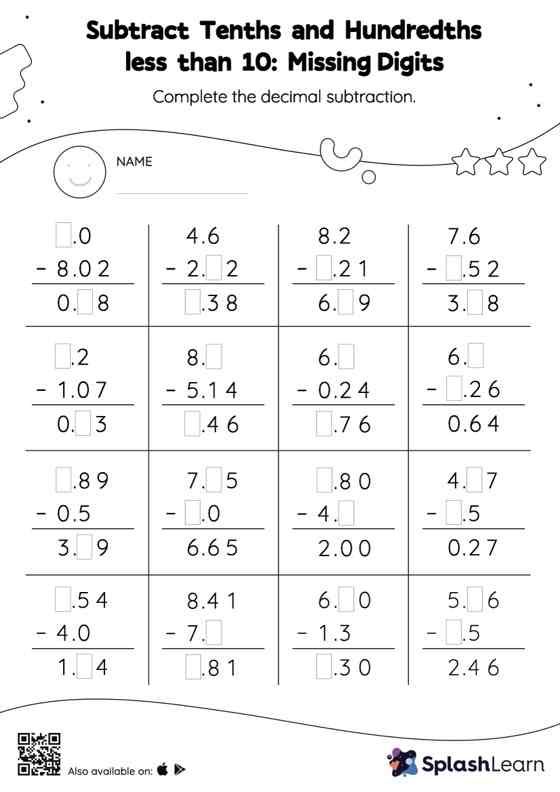# Subtract Tenths and Hundredths less than 10: Missing Digits Worksheet

Home > Subtract Tenths and Hundredths less than 10: Missing DigitsIn this worksheet, students subtract decimals by aligning the decimal points and using zero as a placeholder. They then use the relationship between addition and subtraction to find the missing number in the subtract tenths and hundredths less than 10 worksheet. This worksheet is about practicing the column method in which numbers are written one on top of another. In this method, students use their place value understanding to solve the problems.